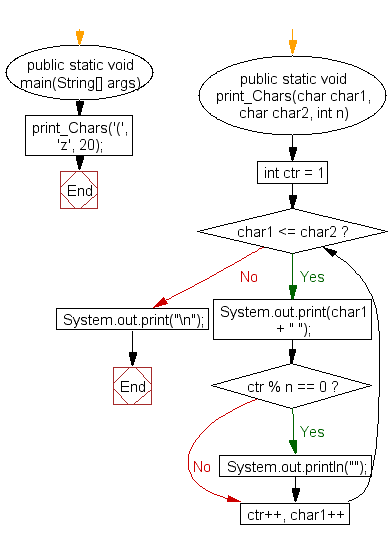﻿ Java exercises: Print characters between two characters - w3resource# Java Method Exercises: Print characters between two characters

## Java Method: Exercise-9 with Solution

Write a Java method to print characters between two characters (i.e. A to P ).

Note: Prints 20 characters per line

Sample Solution:

Java Code:

``````public class Exercise9 {
public static void main(String[] args) {
print_Chars('(', 'z', 20);
}
public static void print_Chars(char char1, char char2, int n) {
for (int ctr = 1; char1 <= char2; ctr++, char1++) {
System.out.print(char1 + " ");
if (ctr % n == 0) System.out.println("");
}
System.out.print("\n");
}
}
```
```

Sample Output:

```( ) * + , - . / 0 1 2 3 4 5 6 7 8 9 : ;
< = > ? @ A B C D E F G H I J K L M N O
P Q R S T U V W X Y Z [ \ ] ^ _ ` a b c
d e f g h i j k l m n o p q r s t u v w
x y z
```

Flowchart:Java Code Editor:

Contribute your code and comments through Disqus.

What is the difficulty level of this exercise?

﻿